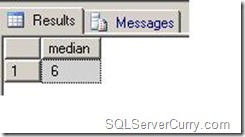### Calculate Median in SQL Server

Median is the numeric value that separates higher half of the list from the lower half. If the list contains odd number of values, median is the number available at the position
(n+1)/2 when numbers are arranged in ascending order. Otherwise it is the average of the numbers at position (n+1)/2 and (n+2)/2. Let us see both these scenarios with examples

Scenario 1: Odd number of values

Create a testing table variable with sample data

declare @table table(number int)
insert into @table
select 3 union all
select 19 union all
select 6 union all
select 1 union all
select 48

--Find number of items in the list
declare @n int
select @n=COUNT(*) from @table

if @n%2=1
select number as median from
(
select ROW_NUMBER() over (order by number) as sno,* from @table
) as t
where sno=(@n+1)/2
else
select avg(number*1.0) as median from
(
select ROW_NUMBER() over (order by number) as sno,* from @table
) as t
where sno in ((@n+1)/2,(@n+2)/2)

OUTPUTScenario 2: Even Number of values

OUTPUT

Row number function is used to assign a serial number based on ascending order of the list. The condition if @n%2=1 checks if the total number of list is odd number and (@n+1)/2 will be the number which indicates the position of the median number and the query takes that number.

If @n%2<>1, the query takes the average of the numbers in the positions (@n+1)/2,(@n+2)/2